## RD Sharma Class 10 Solutions Chapter 5 Arithmetic Progressions Ex 5.1

These Solutions are part of RD Sharma Class 10 Solutions. Here we have given RD Sharma Class 10 Solutions Chapter 5 Arithmetic Progressions Ex 5.1

Other Exercises

Question 1.
Write the first five terms of each of the following sequences whose nth terms are: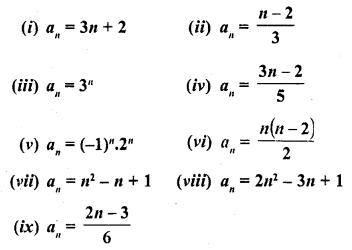Solution: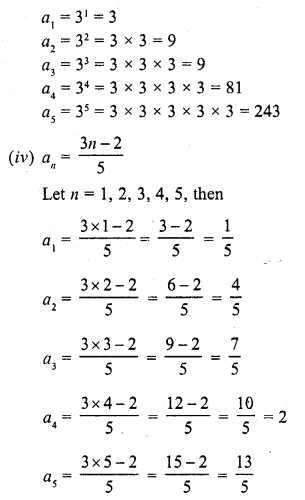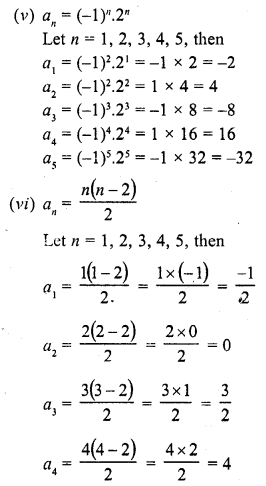Question 2.
Find the indicated terms in each of the following sequences whose nth terms are:Solution:Question 3.
Find the next five terms of each of the following sequences given by :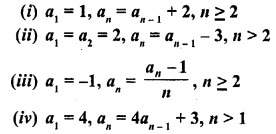Solution: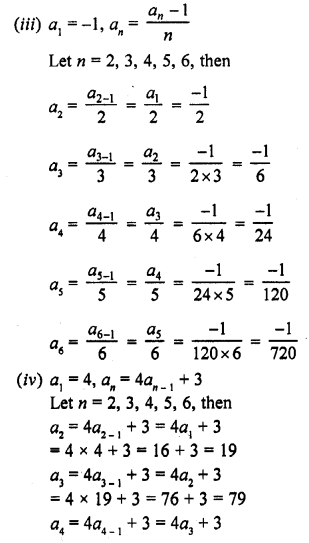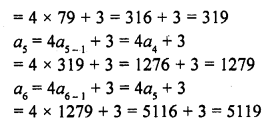Hope given RD Sharma Class 10 Solutions Chapter 5 Arithmetic Progressions Ex 5.1 are helpful to complete your math homework.

If you have any doubts, please comment below. Learn Insta try to provide online math tutoring for you.